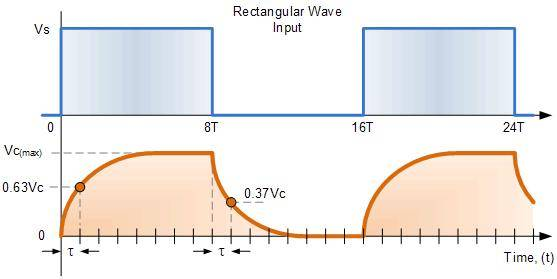# Average of 2 functions

• dimpledur

## Homework Statement

My question pertains to taking the average over a particular period that is composed of 2 functions. For example, from [0, 5ms] the function is defined by 1-e^(-t/1ms)and then by e^(-t/1ms) from [5ms, 10ms].

Will the average from 0-->10 simply be the following:
$$\bar{f}=\frac{1}{5}\int^{5 ms}_{0}{1-e^{-t/1 ms}}+\frac{1}{5}\int ^{5}_{0}{e^{-t/1 ms}}$$

If you look at the bottom graph that is orange, it gives visual representation as to what I am trying to take the average of. One period, two functions. One function for half the period, another function for the last half.Nvm, pretty sure it is as follows:
$$\bar{f}=\frac{1}{10}\int^{5 ms}_{0}{1-e^{-t/1 ms}}+\frac{1}{10}\int ^{5}_{0}{e^{-t/1 ms}}$$
Since the entire period is 10, then both contributions from each function needs to be divided by this number. If I hadn't done this, I would not be considering the other half where each function is zero.